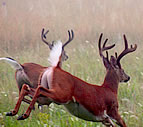×

# decimal

3 ENTRIES FOUND:
1 decimal
/ˈdɛsəməl/
Learner's definition of DECIMAL
mathematics
: based on the number 10
2 decimal /ˈdɛsəməl/ noun
plural decimals
2 decimal
/ˈdɛsəməl/
noun
plural decimals
Learner's definition of DECIMAL
[count] mathematics
: a number that is written with a dot between the part of the number that is equal to 1 or more and the part of the number that is less than 1
called also decimal fraction